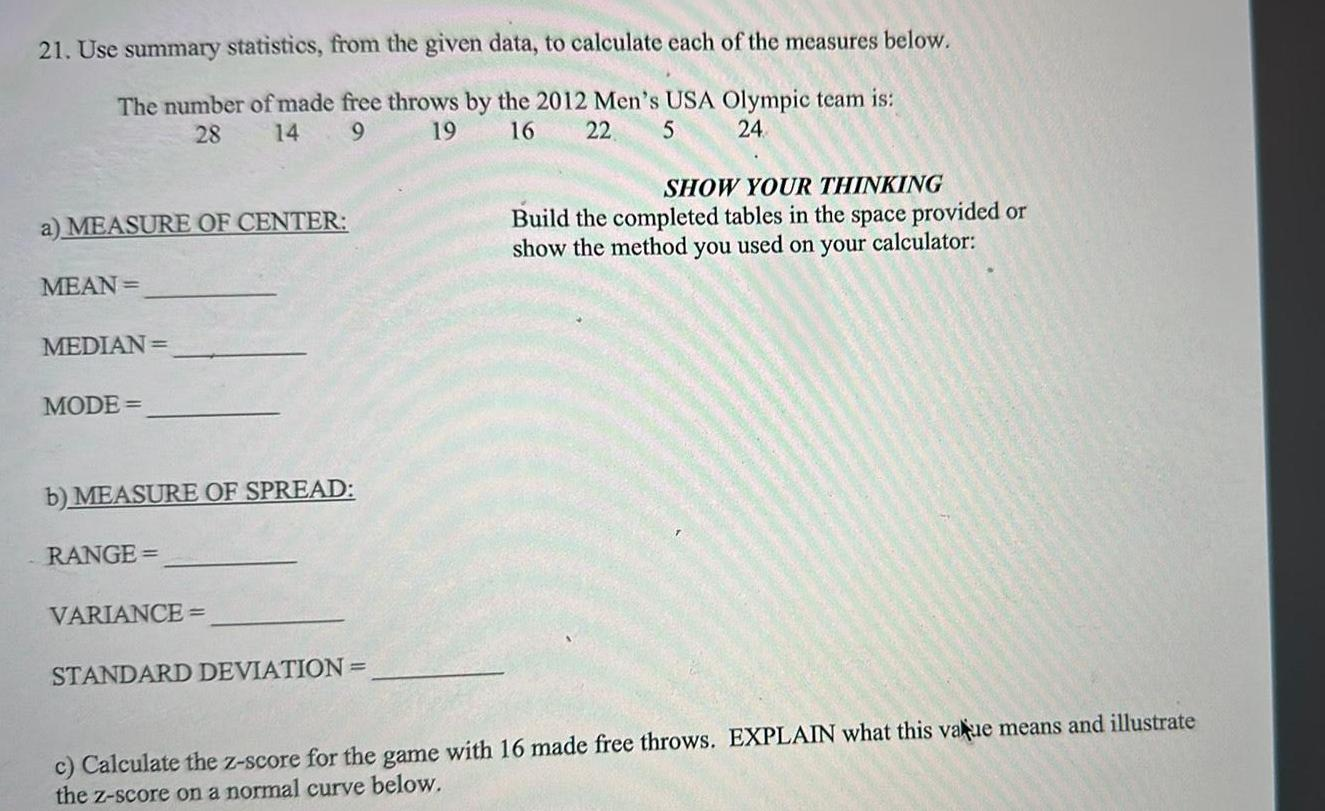Question:

# 21 Use summary statistics from the given data to calculate

Last updated: 9/18/202321 Use summary statistics from the given data to calculate each of the measures below The number of made free throws by the 2012 Men s USA Olympic team is 9 19 28 14 16 22 5 24 a MEASURE OF CENTER MEAN MEDIAN MODE b MEASURE OF SPREAD RANGE VARIANCE STANDARD DEVIATION SHOW YOUR THINKING Build the completed tables in the space provided or show the method you used on your calculator c Calculate the z score for the game with 16 made free throws EXPLAIN what this value means and illustrate the z score on a normal curve below